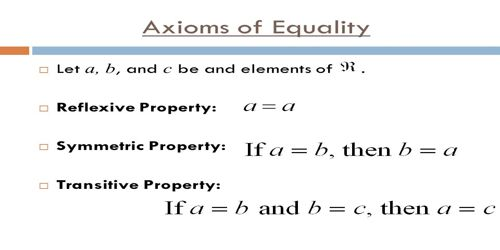Mathematic

# Symmetric AxiomAn Axiom is a mathematical statement that is assumed to be true. There are four rearrangement axioms and two rearrangement properties of algebra. n mathematics, equality is a relationship between two quantities or, more generally two mathematical expressions, asserting that the quantities have the same value, or that the expressions represent the same mathematical object. The equality between A and B is written A = B, and pronounced A equals B. The symbol “=” is called an “equals sign”. Thus there are three kinds of equality, which are formalized in different ways.

• Two symbols refer to the same object.
• Two sets have the same elements.
• Two expressions evaluate to the same value, such as a number, vector, function or set.

These may be thought of as the logical, set-theoretic and algebraic concepts of equality respectively.

Symmetric Axiom: Numbers are symmetric around the equals sign. If a = b then b = a. This is the second axiom of equality. It follows Euclid’s Common Notion One: “Things equal to the same thing are equal to each other.”

The symmetric property of equality states: if a = b, then b = a. In short, with the symmetric property, we can take the left-hand side of the equation (a) and move it to the right-hand side, while taking the right-hand side of the equation (b) and moving it to the left-hand side.

The symmetric property may not seem like much, but it is important. This property allows you to write either x = 5 or 5 = x on your quiz and have either one be the correct answer. You may not have seen the symmetric property used often in arithmetic classes, but it is there as well. We’ll look at arithmetic and algebra examples next.

If x = y then y = x (x = y and y = x are the same thing except x and y are flipped).

If 2x – 4= 18 + x2 then 18 + x2 = 2x – 4

Information Source